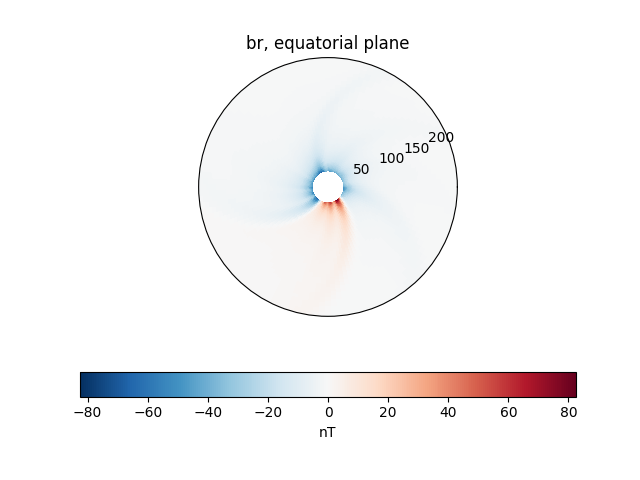# Changing the units of a plot

This example shows how to change the units of data. This is helpful if you want to plot data in units differently to how it is stored in a a model.

```from psipy.model import MASOutput
from psipy.data import sample_data

import astropy.units as u
import matplotlib.pyplot as plt
```

Next, load a set of MAS output files. You will need to change this line to point to a folder with MAS files in them.

```mas_path = sample_data.mas_helio()
model = MASOutput(mas_path)
```

The units of each within `model` can’t be changed, but we can get individual variables and change their units before plotting

```br = model['br']
print(f'Old unit: {br.unit}')
br.unit = u.nT
print(f'New unit: {br.unit}')
```

Out:

```Old unit: G
New unit: nT
```

Plot

```cbar_kwargs = {'orientation': 'horizontal'}
ax = plt.subplot(projection='polar')
br.plot_equatorial_cut(ax=ax, cbar_kwargs=cbar_kwargs)

plt.show()
```Total running time of the script: ( 0 minutes 0.198 seconds)

Gallery generated by Sphinx-Gallery